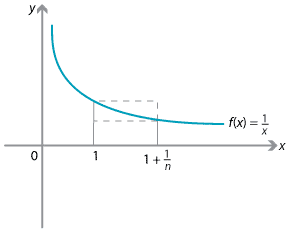### Finding limits using areas

One beautiful extension of the pinching theorem is to bound a limit using areas.

We begin by looking at the area under the curve $$y=\dfrac{1}{x}$$ from $$x=1$$ to $$x=1+\dfrac{1}{n}$$.Detailed description

The area under the curve is bounded above and below by areas of rectangles, so we have

$\dfrac{1}{n} \times \dfrac{1}{1+\dfrac{1}{n}}\leq \int_1^{1+\frac{1}{n}} \dfrac{1}{x}\, dx \leq \dfrac{1}{n} \times 1.$

Hence

$\dfrac{1}{1+n} \leq \log_e\Bigl(1+\dfrac{1}{n}\Bigr) \leq \dfrac{1}{n}.$

Now multiplying by $$n$$, we have

$\dfrac{n}{1+n} \leq n\,\log_e\Bigl(1+\dfrac{1}{n}\Bigr) \leq 1.$

Hence, if we take limits as $$n\to \infty,$$ we conclude by the pinching theorem that

\begin{align*} n\log_e\Bigl(1+\dfrac{1}{n}\Bigr) \to 1 \ &\implies \ \log_e\bigl(1+\dfrac{1}{n}\Bigr)^n \to 1\\ &\implies \ \Bigl(1+\dfrac{1}{n}\Bigr)^n\to e \qquad (!!) \end{align*}

That is,

$\lim_{n\to \infty} \Bigl(1+\dfrac{1}{n}\Bigr)^n = e.$

Next page - History and applications - Paradoxes of the infinite